# The Golden Ratio and Fibonacci

We’re looking at the Fibonacci sequence, and have seen connections to a number called phi (φ or $$\phi$$), commonly called the Golden Ratio. I want to look at some geometrical connections and other interesting facts about this number before we get back to the Fibonacci numbers themselves and some inductive proofs involving them.

## The Golden Rectangle and Fibonacci

The golden ratio, $$\phi$$, which goes back at least to ancient Greece, has also been called the “golden mean” (because it’s a special “middle”), the “golden section” (because it is a special way of “cutting” a segment), the “divine proportion” (because it was considered perfect), and “extreme and mean ratio” (as an explicit description). Specifically, it is the ratio between two parts a and b of a segment such that it is also the ratio between the whole and one of the parts: $$\frac{a}{b}=\frac{a+b}{a}$$

By the way, the terms “golden section” and “golden ratio” were not used until the 1800’s, and the symbol $$\phi$$ was only introduced around 1910.

Euclid defined it this way:

A straight line is said to have been cut in extreme and mean ratio when, as the whole line is to the greater segment, so is the greater to the less.

Here is a “golden rectangle”, whose sides are in the golden ratio: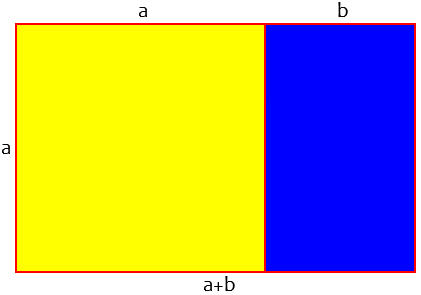We have divided the horizontal line into parts a and b so that the rectangles with ratios a:b [blue] and (a+b):a [whole] are similar.

The Relation of the Golden Ratio and the Fibonacci Series

I am trying to figure out why the Golden Ratio and the Fibonacci series are related.  I have figured out that the ratio of a number in the Fibonacci series over the previous becomes increasingly closer to the golden ratio, but I have no idea how to relate that occurrence to some mathematical reason or logic. I would appreciate any help.

(By the way, it is common to use the word “series”, which properly refers to an infinite sum, instead of “sequence”, which is the proper term for a mere list of numbers. We don’t always correct that! The Fibonacci sequence is also often just called “the Fibonacci numbers”.)

We’ve already seen, as this student has, that the ratio of Fibonacci numbers approaches $$\phi$$; we’ve even seen a proof, and will see another later. But is there a bigger way to understand it? Doctor Ethan answered:

You have done the sticky part. Now let's look at the beautiful part.

You may have noticed that if you have a Golden rectangle and you cut off a square with side lengths equal to the shorter rectangle side, then what remains is another Golden rectangle.

This could go on forever. You can just keep cutting off these big squares and getting smaller and smaller Golden rectangles.

Here are several repetitions of the process: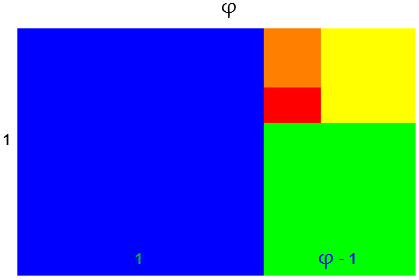The red rectangle is similar to the original whole.

The idea with the Fibonacci series is to do the same thing in reverse.

Here what you do is start with a square (1 by 1), find the longer side, and add a square of that size to the whole thing to form a new rectangle.

So when we start with a 1 by 1 square the longest side is one, so we add another square to it.  Now we have a 2 by 1 rectangle

Then the longest side is 2, so we connect a 2 by 2 square to our 2 by 1 rectangle to get a 3 by 2 rectangle.  This continues, and the sides of the rectangle will always be a successive Fibonacci number.  Eventually the rectangle will be very close to a Golden Rectangle.

If we started with my red rectangle above and added squares to the longer side, we would get the same figure, just in reverse order. What if, instead of starting with a golden rectangle, we started with a square? Here is the resulting figure: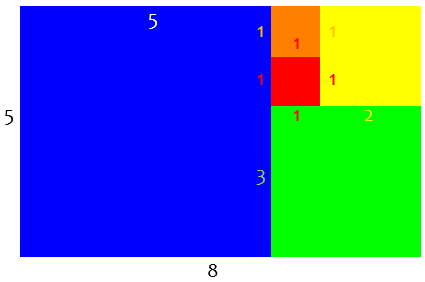You can see that the sides of the successive squares are 1, 1, 2, 3, 5, … , which is the Fibonacci sequence; and the ratios of the rectangles are 1:1, 2:1, 3:2, 5:3, 8:5, … . And as we proceed, the “error” in the rectangle we started with has less and less effect, so that our rectangles become more and more like the golden rectangle.

While we’re looking at rectangles, suppose we show the circular arcs I drew with a compass to find the new length at each step: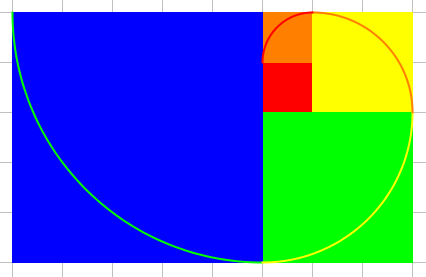This Fibonacci spiral is similar to one called the golden spiral, but is not identical.

## A little history

Here is another 1996 question:

Golden Rectangle History

Can you tell me anything about the history of the golden rectangle? When was it discovered and by whom?

There are entire books written about the golden rectangle or the golden section.  Some books concentrate on the applications of the golden section or ratio to architecture.  Try any good book on the history of mathematics.

Another source is Sir Thomas L. Heath's edition of Euclid's Elements.  He gives many historical comments in footnotes and other discussion.  For example, in vol. 1, page 137 of the Dover paperback edition, Heath mentions that Plato refers to the golden section (closely related to the golden rectangle) and Euclid includes the construction in proposition 11 of Book II of his Elements.  Heath also says that the idea is most probably Pythagorean, which means that it was known ca. 500 BC.  I doubt if it is known or will ever be known exactly who discovered the golden section.

Keep in mind that it wasn’t called the golden ratio or golden section yet! And there are a lot of myths about this number as well; its use in ancient architecture probably wasn’t quite what many people think, and it is “found” in many places in nature where it really doesn’t exist.

As Heath says, "As the solution of this problem [see below] is necessary to that of inscribing a regular pentagon in a circle, we must necessarily conclude that it was solved by the Pythagoreans, or, in other words, that they discovered the geometrical solution to the quadratic equation a(a-x)=x^2."

We’ll see a pentagon soon.

Proposition 11 of Book II is: To cut a given straight line so that the rectangle contained by the whole and one of the segments is equal to the square on the remaining segment.

If AB is the given line, we want to cut it at H so that AB*BH = AH*AH.  If AB has length 1 and AH = x, then Prop. 11 is, in modern terms, x^2+x-1 = 0.  The positive root is (-1+sqrt(5))/2, which is approx 0.62.  This is the golden ratio, on which the golden rectangle is based.

Euclid’s construction is found in Book II Prop 11 (as just stated), and also in Book VI Prop 30 (described as “extreme and mean ratio”). Here is the figure from the former: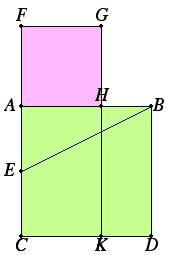The pink square AFGH has the same area as the rectangle on the right, BDKH. Observe that the ratio Doctor Jerry stated is what we have called $$\Phi=\frac{1}{\phi}$$. For an aside on that, continue:

## Two phi‘s for the price of one?

phi vs. Phi - a Coincidence?

Ancient and modern architecture reflect the 'golden ratio' (1.618 length to width) and this number is remarkably close to phi (.618...) seen in nature for leaf dispersions, etc. Is this just a coincidence?

This student has evidently seen the first number in connection with geometry and architecture, and the second in connection with nature. I answered, going along with his terminology:

It's more than just coincidence: the golden ratio (as you define it) is phi's twin, "Phi," where

Phi = (sqrt(5) + 1)/2 = 1.618...

phi = (sqrt(5) - 1)/2 = 0.618...

Phi = 1/phi

Phi = 1 + phi

The latter facts together give the definition of the golden ratio:

x = 1/x + 1

This equation (equivalent to x^2 - x - 1 = 0) is satisfied by both Phi and -phi, which therefore can be called the _golden ratios_. Since they are reciprocals, either could just as well be given that name.

I’ve been using the upper case ($$\Phi$$) and lower case ($$\phi$$) forms the other way around, which is perhaps more standard. But as I said, it doesn’t make much difference.

Together, these are used in the formula for the Fibonacci sequence:

F[n] = (Phi^n - (-phi)^n) / sqrt(5)

It gets confusing after a while, doesn’t it?

## The Golden Triangle

Here is a question from 1999 about the Golden Triangle:

Golden Triangle: What is It?

I'm doing a project for math where I have to answer questions using the Internet. I have gone to many different Web sites trying to figure out what the Golden Triangle is, but I cannot find it. It has to do with math but so far all my results have something to do with bicycles. Please help me find out what the Golden Triangle is. Thank you.

Hi, Robin,

Suppose we have a triangle ABC, such that <A = <B = 72 degrees (< means angle) and <C = 36 degrees. Such a triangle is known as the Golden Triangle.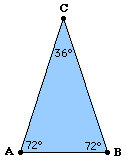What makes this special? And can you see the connection to the golden ratio?

Let D be the point on BC, such that AD is the angle bisector of <A. Then triangle ABD is again a Golden Triangle.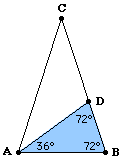This is true simply because 72 is twice 36! In fact, that is how the angles in this triangle were chosen: We wanted the base angles to be twice the apex angle; if the latter is x, then $$2x+2x+x=180$$, so that $$x=\frac{180}{5}=32$$.

Now, what can we say about the side lengths? The fact that triangles BAD and ADC are isosceles lets us mark three segments equal: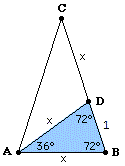And since triangles BAD and BCA have the same base angles, they are similar and we can write a familiar proportion:

When we let lengths AB = AD = CD = x and BD = 1, then we find:

AB : BD  =  BC : AB
x : 1   = (x+1) : x

This can be rewritten to:

x^2 = x + 1
x^2 -x - 1 = 0

The two solutions for x are x = 1/2 +/- sqrt(5)/2. Since AB > BD, in this case we must have x = 1/2 + sqrt(5)/2.

The number 1/2 + sqrt(5)/2 is known as the Golden Ratio, or Golden Mean. So BC : AB is this famous ratio; that's why this triangle is called a Golden Triangle.

But there’s more!

As an example of the appearance of Golden Triangles: the outside triangles of a pentagram are Golden Triangles.


Here is a pentagram (regular five-pointed star) showing the five golden triangles it contains:When we attach to AC and BC two triangles that are congruent to triangle ACD, we find a regular pentagon.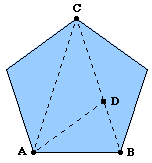This is true simply because the angles add up right. And this connection to the pentagon may be the main reason Euclid found this ratio worth exploring.

## More on the golden triangle

A student in 2001 had a wrong impression of the meaning of the name:

Golden Triangle: An Isosceles Triangle

I have talked to my Geometry teacher about whether there is such a thing as a Golden Triangle, and he says no, but my Algebra Honors teacher tells me that there is, and it is a right triangle with the ratio 3:4:5, and any of the multiples, like if you multiply by 2, 6:8:10.

I want to know if it exists. I am positive it was in my textbook from Algebra 1.  Please help solve the issue.

Hi Grayson,

What I've heard called a golden triangle is more like a golden rectangle. A golden rectangle has the property that if you cut off a square from it, the remaining rectangle is the same shape as the original rectangle, only smaller. Its sides are in the ratio 1 : (1 + sqrt(5))/2, or about 1 : 1.618033.

The golden triangle is an isosceles triangle. It has the property that, if you bisect one of the base angles, one of the triangles you cut off is similar to the original triangle. If its base is 1 unit long, the two equal sides are each (1 + sqrt(5))/2 units long, the same ratio as the golden rectangle.

Golden triangles can be found in pentagons.  In a regular pentagon ABCDE, the triangle ACD is a golden triangle: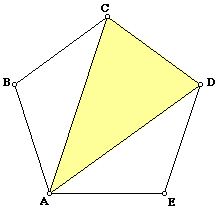## Golden ratio as a limit

Let’s get back to Fibonacci, with this question from 1998:

The Golden Ratio

I know that the limit of the ratios of the Fibonacci sequence is the golden mean, but I would like to see a proof of this.

We’ve previously touched on this, but there’s more to be said. Doctor Rob replied to Karen:

The Fibonacci numbers satisfy the recursion

F(0)   = 0,
F(1)   = 1,
F(2)   = 1,
F(n+1) = F(n) + F(n-1),

for all n > 1.  Divide both sides by F(n), and rearranging:

F(n+1)/F(n) = 1 + 1/[F(n)/F(n-1)].

This, of course, is the definition of the Fibonacci sequence, expressed in terms of ratios.

Now suppose the ratio converges to some limit A.  Take the limit of both sides as n -> infinity:

A = 1 + 1/A,
A^2 - A - 1 = 0,
A = (1 + Sqrt)/2,

the Golden Ratio, after we reject the negative root as not meaningful.

This shows that if the ratio converges to anything, it converges to the Golden Ratio.

The equation here is the equation that defined the golden ratio (and its negative reciprocal).

More work would be needed to properly prove that the sequence does converge, to satisfy a mathematician, but convergence is obvious for practical purposes. (Convergence means that the numbers actually approach some limit at all.)

Alternatively, it can be proved using what we saw last week:

For another approach to show that it converges to the Golden Ratio, you can use the Binet Formula for F(n):

F (n) = (A^n - B^n)/(A - B),
A  = (1 + Sqrt)/2,
B  = (1 - Sqrt)/2,
A + B  = 1,
A - B  = Sqrt.

This is another way to express Binet’s formula, where $$A = \phi$$ and $$B = 1-\phi = -\frac{1}{\phi}$$.

Since |B| < 1, as n gets very large, B^n gets very small, and we find that, as n -> infinity,

lim F(n+1)/F(n) = lim (A^[n+1] - B^[n+1])/(A^n - B^n),
= lim A^[n+1]/A^n,
= lim A,
= A.

Which, of course, is $$\phi$$.

The Binet Formula can be proved by induction.  It also leads to the very interesting

F(n) = Round[A^n/Sqrt],  for all n >= 0,

which tells us that the Fibonacci numbers are almost in geometric progression with common ratio A.

This is because the absolute value of the second term is always less than 1/2 (for positive n), so rounding to the nearest whole number always gives us $$F_n$$. Here are successive values of the two terms $$\frac{\phi^n}{\sqrt{5}}$$ and $$\frac{(1-\phi)^n}{\sqrt{5}}$$, and the actual values:

$$F_1 =0.72361 +0.27639 =1\\ F_2 =1.17082 -0.17082 =1\\ F_3 =1.89443 +0.10557 =2\\ F_4 =3.06525 -0.06525 =3\\ F_5 =4.95967 +0.04033 =5\\ F_6 =8.02492 -0.02492 =8\\ F_7 =12.98460 +0.01540 =13\\ F_8 =21.00952 -0.00952 =21$$

The first term is alternately just below and just above the value of the term, so we can just round it and ignore the second term.

## Golden ratio as a continued fraction

Another amazing representation of phi is the subject of this 1997 question:

Advanced Algebra

My advanced algebra teacher has given us this problem for extra credit. So far no one in the class has gotten it! Can you help?  The problem looks like this:

1 +         1
-----------------
1 +       1
-------------
1 +     1
---------
1 +   1
-----
1 + 1
.
.
.

My teacher said it had something to do with the golden ratio. I turned in an answer of 1 because I thought the bigger the denominator got, the smaller the fraction and the fraction would eventually be = 0.  He said that was wrong. I hope you can help me, not just with the answer but help me to understand the solution!

This form is called an infinite continued fraction, whose value is the limit of the finite continued fractions found by stopping after some number of terms, called convergents. Here are the first few:

$$c_0=1$$

$$c_1=1+\frac{1}{1}=1+1=2$$

$$c_2=1+\frac{1}{1+\frac{1}{1}}=1+\frac{1}{2}=\frac{3}{2}$$

$$c_3=1+\frac{1}{1+\frac{1}{1+\frac{1}{1}}}=1+\frac{1}{\frac{3}{2}}=1+\frac{2}{3}=\frac{5}{3}$$

Look familiar?

To see what this value is, call it x.  So

x = 1+1/(1+1/(1+1/(1+1/1+...))).

Subtract 1 from both sides:

x-1 = 1/(1+1/(1+1/(1+1/1+...))).

"Flip" the numerator and denominator of both sides (invert):

1/(x-1) = 1+1/(1+1/(1+1/1+...)).

But notice that the righthand side is still x, because the fraction continues infinitely (compare with what we had written in the first line).  Therefore,

1/(x-1) = x,

or x(x-1) = 1. From here, it is easy to solve for x, and you will see why the hint was "the golden ratio."

This becomes the now-familiar equation $$x^2-x-1=0$$, whose solutions are $$\phi$$ and $$1-\phi$$; since the convergents are all positive, their limit (assuming it exists) must be positive, so we have our answer: the continued faction is, in fact, the golden ratio.

But there’s more! We can also use a continued square root:

For another related problem, what is the value of

1+Sqrt[1+Sqrt[1+Sqrt[1+Sqrt[1+...]]]]?

(Sqrt[x] means the square root of x.)  Hint: Use the same method as in the previous problem.  If you find the correct answer, prepare to be surprised!

By the same reasoning, if $$x=1+\sqrt{1+\sqrt{1+\sqrt{1+\sqrt{1+\cdots}}}}$$, then $$(x-1)^2=x$$, which simplifies to $$x^2-3x+1=0$$, so that $$\displaystyle x=\frac{3\pm\sqrt{5}}{2}$$ which we encountered last week; this is $$1+\phi=\phi^2$$!

If the expression had been $$x=\sqrt{1+\sqrt{1+\sqrt{1+\sqrt{1+\cdots}}}}$$, we would have $$x^2-1=x$$, whose positive solution would be $$\phi$$.

Now, why is it that your reasoning was flawed? Well, because you are assuming that the value underneath the fraction gets larger and larger, when in fact it does not.  From the solution I have provided, you may realize that what happens is that the stuff under the first fraction is the very same value you wish to seek, and therefore is not infinite! Similarly, in the second problem I posed to you, the stuff under the first square root is the very same value you wish to find.

1.